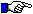## Daquing Wan, University of California, Irvine ``Introduction To Dwork's Conjecture'' February 18, 1999

In this lecture, I shall give an elementary and self-contained introduction to Dwork's conjecture (1973) on the p-adic meromorphic continuation of his unit root L-function L(f,T) attached to a family f: Y->X of algebraic varieties over a finite field of characteristic p. This conjecture is a p-adic extension of the Weil conjecture (1949) from a single variety (or a family of zero-dimensional varieties) to an arbitrary family of varieties.

When f is a family of zero-dimensional varieties (i.e., f is a finite map), the unit root L-function L(f, T) becomes the zeta function Z(Y,T) of the variety Y. The zeta function Z(Y,T) is a generating function which counts the number of rational points on Y. The zeta function Z(Y,T) is a rational function as conjectured by Weil and first proved by Dwork (1960) using p-adic analytic method. The key step in Dwork's proof is to show that Z(Y,T) is p-adic meromorphic. The zeros and poles of the zeta function satisfy a suitable Riemann hypothesis as also conjectured by Weil but proved by Deligne (1974-1980) using etale cohomology.

When f is a family of positive-dimensional varieties, the unit root L-function L(f, T) is no-longer a rational function and the situation is much more mysterious. But Dwork conjectured that the L-function is p-adic meromorphic. The simplest example is the universal family f_E of elliptic curves. In this case, the L-function L(f_E,T) is known to be p-adic meromorphic (Dwork, 1971). However, even in the elliptic family case, very little is known about the absolute values of the zeros of L(f_E,T), namely, the p-adic Riemann hypothesis for L(f_E,T), which contains important arithmetic information about modular forms such as the Gouvea-Mazur conjecture and the p-adic Ramanujuan-Peterson conjecture.A note from the colloquium chair: I highly recommend the Dwork memorial article which was written for the March, 1999 Notices by Nick Katz and John Tate.